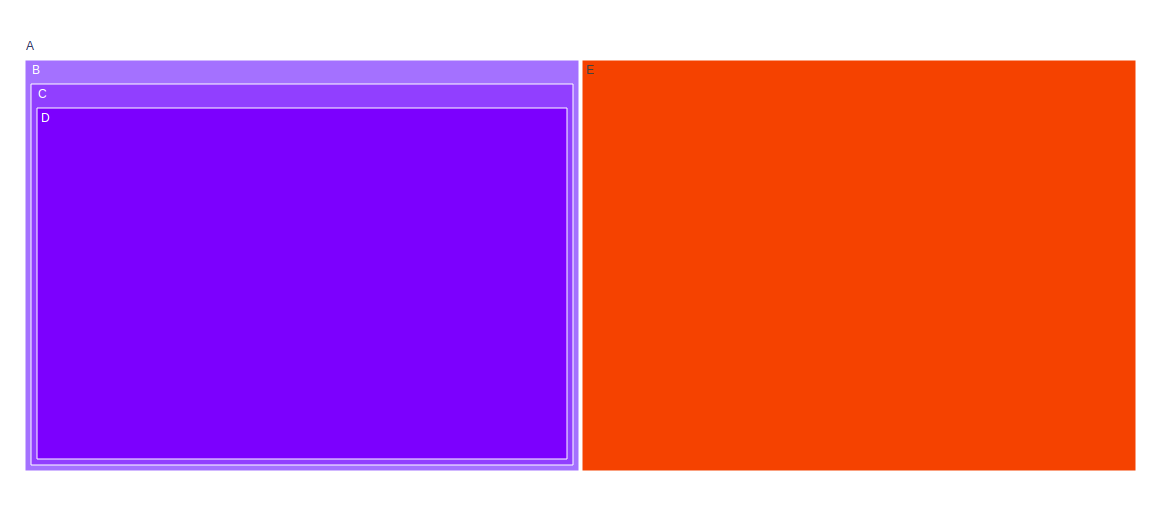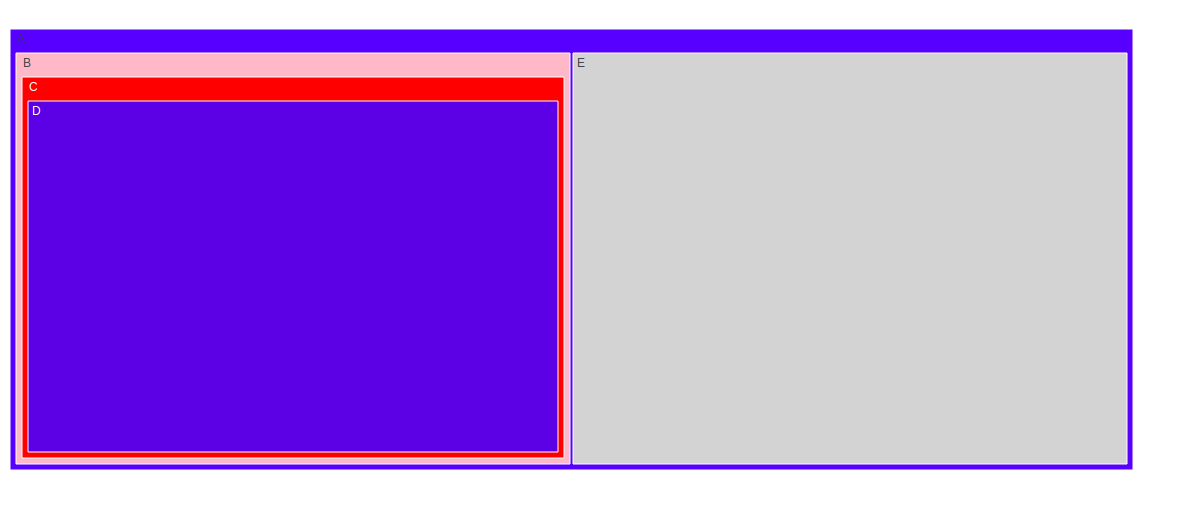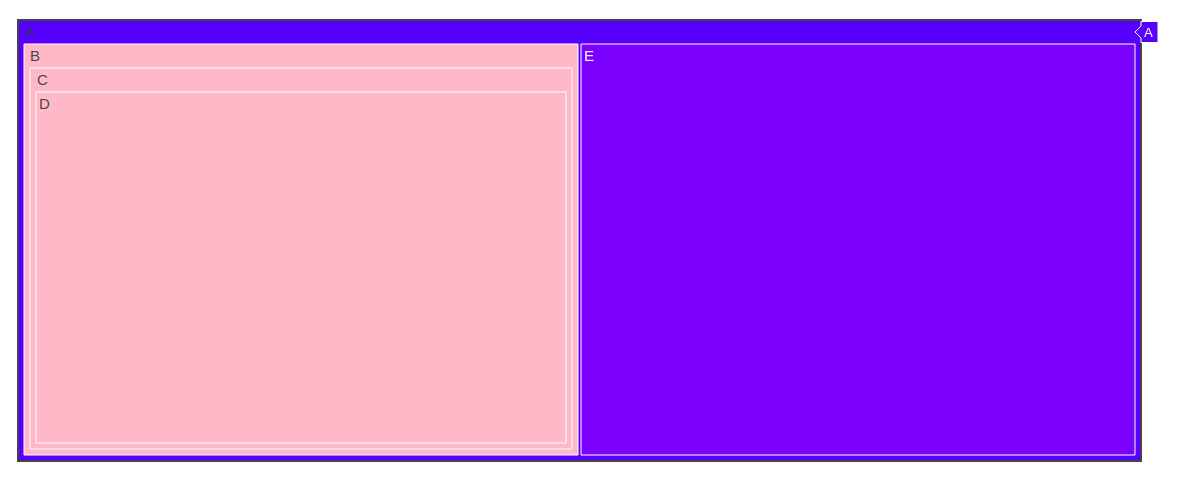# Treemap using graph_objects class in plotly

Plotly is a Python library which is used to design graphs, especially interactive graphs. It can plot various graphs and charts like histogram, barplot, boxplot, spreadplot and many more. It is mainly used in data analysis as well as financial analysis. plotly is an interactive visualization library.

## Treemap using graph_objects class

Treemapping is a data visualization procedure which can be used to demonstrate graded data using enclosed rectangles. Data is organized as branches and sub_branches by representing using rectangles, dimensions and plot color.Tree map chart helps in distinguishing the categories between data values apparently.

Syntax: class plotly.graph_objects.Treemap(arg=None, branchvalues=None, count=None, customdata=None, hoverinfo=None, **kwargs)

Parameters:

arg: dict of properties compatible with this constructor or an instance of plotly.graph_objects.Treemap

branchvalues: Determines how the items in values are summed.

count:  Determines default for values when it is not provided, by inferring a 1 for each of the “leaves” and/or “branches”, otherwise 0.

customdata:  Assigns extra data each datum. This may be useful when listening to hover, click and selection events. Note that, “scatter” traces also appends customdata items in the markers DOM elements

hoverinfo: Determines which trace information appear on hover. If none or skip are set, no information is displayed upon hovering. But, if none is set, click and hover events are still fired.

Example:

## Python3

 `import` `plotly.graph_objects as go ` ` `  `fig ``=` `go.Figure(go.Treemap( ` `    ``labels ``=` `[``"A"``, ``"B"``, ``"C"``, ``"D"``, ``"E"``], ` `    ``parents ``=` `["``", "``A``", "``B``", "``C``", "``A"]  ` `)) ` ` `  `fig.show()`

Output:## Set Color of Treemap Sectors

To change the color sector in treemap there are three different ways:

• marker.colors
• colorway
• colorscale

Example:

## Python3

 `import` `plotly.graph_objects as go ` ` `  `fig ``=` `go.Figure(go.Treemap( ` `    ``labels ``=` `[``"A"``, ``"B"``, ``"C"``, ``"D"``, ``"E"``], ` `    ``parents ``=` `["``", "``A``", "``B``", "``C``", "``A"], ` `    ``marker_colors ``=` `[``"blue"``, ``"pink"``, ``"red"``,  ` `                 ``"royalblue"``, ``"lightgray"``, ] ` `)) ` ` `  `fig.show()`

Output:## Changing text fontsize

In plotly, the labels can be remain of same size by using uniformtext  layout parameter.The font size are set by minsize attributes, and mode attributes are set for labels cannot fit with the desired fontsize: either hide them or show them with overflow.

## Python3

 `import` `plotly.graph_objects as go ` ` `  `fig ``=` `go.Figure(go.Treemap( ` `    ``labels ``=` `[``"A"``, ``"B"``, ``"C"``, ``"D"``, ``"E"``], ` `    ``parents ``=` `["``", "``A``", "``B``", "``C``", "``A"], ` `marker_colors ``=` `[``"blue"``, ``"pink"``] ` `)) ` ` `  `fig.update_layout(uniformtext ``=` `dict``(minsize ``=` `15``, ` `                                   ``mode ``=``'hide'``)) ` ` `  `fig.show()`

Output:My Personal Notes arrow_drop_upIf you like GeeksforGeeks and would like to contribute, you can also write an article using contribute.geeksforgeeks.org or mail your article to contribute@geeksforgeeks.org. See your article appearing on the GeeksforGeeks main page and help other Geeks.

Please Improve this article if you find anything incorrect by clicking on the "Improve Article" button below.

Article Tags :

Be the First to upvote.

Please write to us at contribute@geeksforgeeks.org to report any issue with the above content.